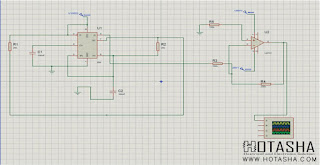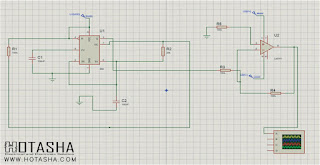# To make a square wave generator with adjustable frequency and amplitude

Square wave can be defined as a non sinusoidal periodic waveform that can be represented as an infinite summation of sinusoidal waves. It has an amplitude alternate at a regular frequency between fixed minimum and maximum value with the same duration. Square wave generator are generally used in electronics and in signal processing. The square wave is the special case of rectangular wave. The square wave generator is just like a Schmit trigger circuit in which the reference voltage for the comparator depends on the output voltage. It is also said to be astable multivibrator.

Introduction

Square wave generator can be constructed using Schmitt trigger inverters like TTL. It is the easy way to make a basic astable waveform generator. While producing clock or timing signals, this astable multivibrator produces a square wave generator waveform that switches between HIGH and LOW .

As we know output of a Schmitt inverter is the opposite or inverse to that of its input. By giving hysteresis it can change state at different voltage levels. It uses a Schmitt trigger action that changes state between an upper and lower threshold level as the input voltage signal, increases and decreases about the input terminal. Here upper threshold level sets output and the lower threshold level resets the output.

This simple square wave generator circuit consists of a single TTL 74LS14 Schmitt inverter logic gate with a capacitor connected between its input terminal and ground and the positive feedback required for the circuit to oscillate being provided by the feedback resistor.

Assume that the charge across the capacitor plates is below the Schmitt’s lower threshold level. Therefore makes the input to the inverter at a logic ZERO level, resulting in a logic ONE output level.

Equipments

2. Connecting Wires
3. Resistor(10k,100k,10k,200k)
4. Capacitor(100nF,10uF,1uF)
5. 555 Timer
6. LM 741 Op-amp
7. Dc voltage(10V,15V,-15V)

Working Principle

The 555 timer requires a power supply voltage of 4.5-16V. We connect this voltage to the VCC pin, pin 8, and we connect GND, pin 1, to ground.

The only other pins we use are the trigger pin, the output pin, the reset pin, and the threshold pin.

Pin 2 is the trigger pin. It works like a starter pistol to start the 555 timer running. The trigger is an active low trigger, which means that the timer starts when voltage on pin 2 drops to below 1/3 of the supply voltage. When the 555 is triggered via pin 2, the output on pin 3 goes high.

Pin 3 is the output pin. 555 timer's output is digital in nature. It is either high or low. The output is either low, which is very close to 0V, or high, which is close to the supply voltage that's placed on pin 8. The output pin is where you would connect the load that you want the 555 timer to power. This may be an LED, for instance.

Pin 4 is the reset pin. This pin can be used to restart the 555 timer's timing operation. This is an active low input, just like the trigger input. Thus, pin 4 must be connected to the supply voltage of the 555 timer to operate. If it is momentarily grounded, the 555 timer's operation is interrupted and won't start again until it's triggered again via pin 2.

Pin 6 is the threshold pin. The purpose of this pin is to monitor the voltage across the capacitor that's discharged by pin 7. When this voltage reaches 2/3 of the supply voltage (VCC), the timing cycle ends, and the output on pin 3 goes low.

A 555 timer chip, the maximum voltage that a 555 timer can withstand is 18V. Therefore, we use 10V for the supply DC voltage for this circuit. By attaching a potentiometer to the 10V, we can create an adjustable power source, so that we can alter the amplitude of the output signal. If the potentiometer is turned so that it is offering full resistance, the voltage fed to the 555 timer is about 10V. This gives the maximum amplitude that the output signal can be. If we lower the resistance of the potentiometer, the voltage fed to the 555 timer decreases. This decreases the amplitude of the output signal.

For this circuit, we use a 100Ω potentiometer to allow for amplitude adjustment. A potentiometer leads to abrupt changes in amplitude, which makes for poor amplitude adjustment.

This voltage is fed into the V+ pin of the 555 timer, which is pin 8.

So again, if the potentiometer is offering full resistance, the amplitude is at its maximum amplitude. As we lower the resistance of the potentiometer, the amplitude decreases and decreases until the voltage feeding into pin 8 is so low, that no signal is output.

10V is, fed into pin 4 of the 555 timer. Pin 4 is the reset pin of the 555 timer. This pin is active low, which means it is triggered when fed a voltage near ground or 0V. Therefore, this pin must remain connected to a positive voltage source in order for the circuit to work.

So, this fulfills the power for the circuit.

Besides the amplitude, which we have covered, we now get into the frequency aspect of the circuit, how the frequency of the signal. And the frequency of the output is determined by the potentiometer R1 and capacitor C1. These form an RC network that determine the frequency of the output signal. The product of RC is equal to the time period of the output signal. By decreasing the value of the resistor, we decrease the time period, which creates a signal with a higher frequency. By increasing the value of the resistor, we increase the time period, which creates a signal with a lower frequency. The potentiometer allows us to change the frequency by changing the value of the RC network.

The value of the capacitor C1 that we have chosen is 100nF. If we want even a greater frequency that can go lower, we can choose a lower value capacitor such as in the picofarads range. This extends the frequency range of the circuit, at the high end. The smaller of a capacitor we use, the higher the frequency. For example, a 10nF capacitor causes even a faster frequency.

The potentiometer value that we use for the RC network is a 100k potentiometer. We can also use a 10MΩ potentiometer if we want to extend the frequency range of the circuit, at the low end. The greater of a resistance value you use, the lower frequency can extend. For example, with a 100kΩ potentiometer, the frequency will be just a few hertz on the lower end of the frequency range when the potentiometer is offering its full resistance.

The 555 timer is a very good chip that is able to produce and output very good, high-quality square wave signals. And depending on the values you use, we should be able to get any square wave of any frequency you desire. The 555 timer chip does have a ceiling of frequency but it is several megahertz. So until you exceed this, the circuit should be able to do what we want it to accomplish.

So this is an adjustable square wave generator circuit.Circuit diagram of Square wave generator with adjustable frequency and amplitude.Simulation diagram of Square wave generator with adjustable frequency and amplitude.Circuit diagram of Square wave generator with adjustable frequency and amplitude using variable resistor.Simulation diagram of Square wave generator with adjustable frequency and amplitude using variable resistor.

Future Work

The applications of Astable multivibrators involve in radio gears to transmit and receive radio signals and also in time, morse code generators and some systems which require a square wave like analog integrated circuit and TV broadcasts.

Conclusion

These multivibrators are changes between one stable state to another continuously. This permits multivibrators to power themselves & achieve work at a constant rate without impact from any external forces or actions. Also, these multivibrators are low cost to produce, and simple to design

These multivibrators do not transfer the whole o/p signal to the i/p. This is due to resistance in the circuit, nonexistence of a totally closed loop at the o/p terminals, and the leaning of one transistor/capacitor to absorb energy at a somewhat different rate than the other. Although the amplifier restores the lost energy when it amplifies the signal, the signal will finally be too small to be of any use.

This is all about astable multivibrator using 555 timer, advantages,disadvantages and applications.We hope that you have got a better understanding of this concept, furthermore any doubts regarding this topic please give your suggestions in the comment section below.

Edited By
Fariha Alam
Electrical & Electronic Engineering (EEE)
Chittagong University of Engineering and Technology (CUET)